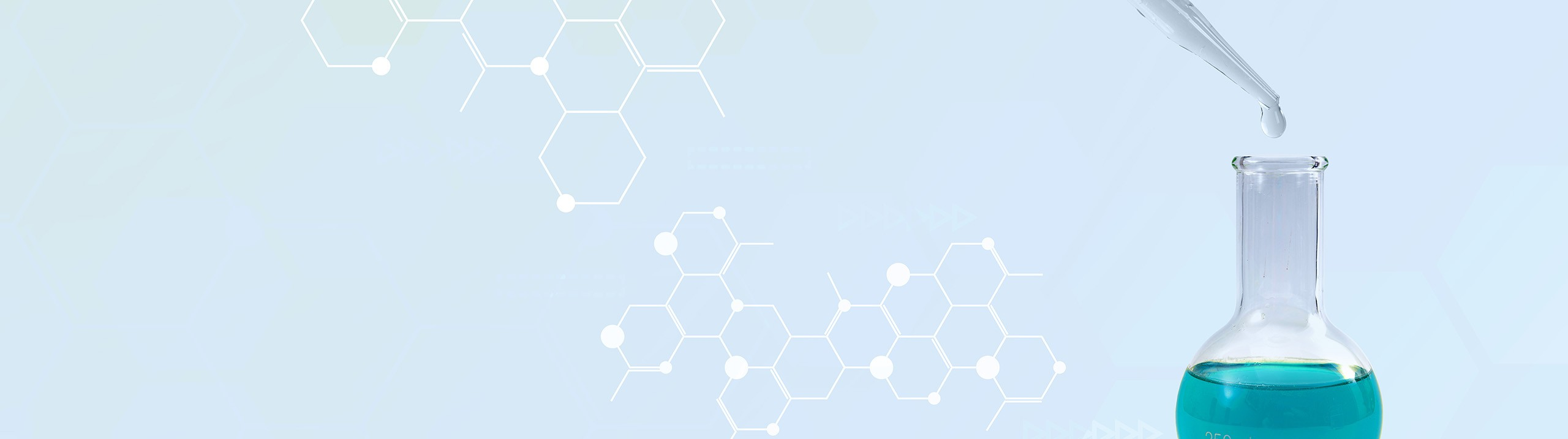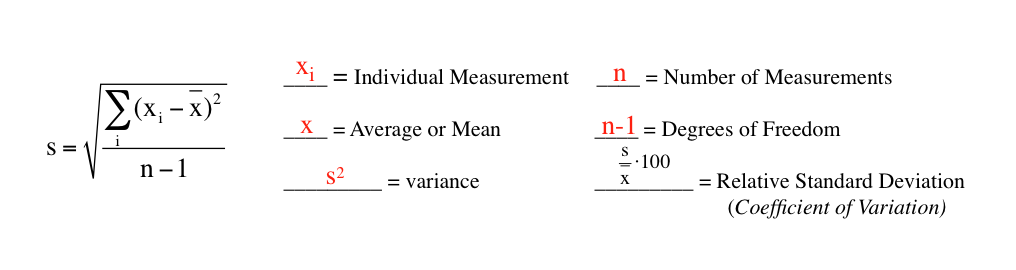## Analytical Chemistry

Learn the toughest concepts covered in your Analytical Chemistry class with step-by-step video tutorials and practice problems.

4 & 5. Statistics, Quality Assurance and Calibration Methods

# Mean Evaluation

Standard Deviation can be used to determine how precise a series of calculations are in relation to one another.

Mean Evaluation
1
example

## Mean Evaluation2m
Play a video:
So here we're gonna say that our standard deviation measures how close data results are in relation to the mean or average value. So basically the smaller standard deviation is the more precise, your measurements will be in relation to the mean or average value. So here the formula for standard deviation is s which stands for standard deviation equals the square root. And here we have the summation of our measurements minus our average squared divided by n minus one. In terms of this equation, we're going to say here that X I here represents an individual measurement that we're undertaking in terms of our dataset, we're going to say that our average or mean value is represented by X. With the line on top, variance is just our standard deviation squared. Later on, when we get more into statistical analysis will see that the f test has a close relationship to the variants of our calculations. Next we have and which represents our numbers of measurements and and -1 represents our degree of freedom. Finally, we have our relative standard deviation also called R coefficient of variation. That is just our standard deviation divided by our meaner average value, times 100. So at some point we're gonna run into using one of these um variables in terms of the standard deviation equation. So just remember the smaller standard deviation is the more precise all your measurements are within your data set. Now your measurements can be precise but that doesn't necessarily mean they will be accurate. Remember accuracy is how close you are to a true value. Your measurements themselves may be close to one another, but still far off from the actual true value. So the accuracy may not be good. Now that you've known the basics of standard deviation, we'll take a look at the example left below, so click on the next video and see how I approach this question, which deals with standard deviation.2
example

## Mean Evaluation4m
Play a video: